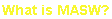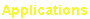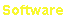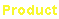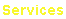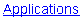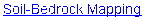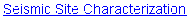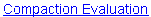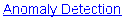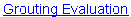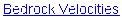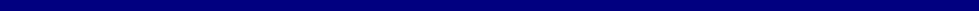e-mail: contact@masw.com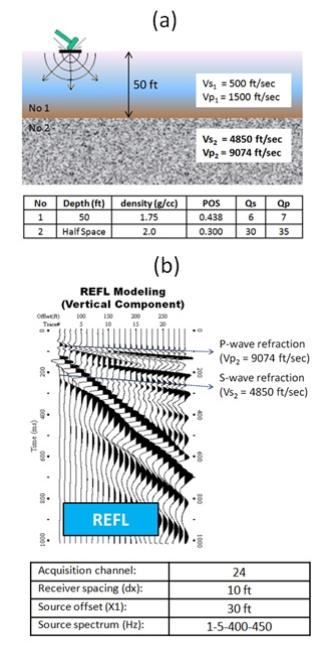MASW Analysis of Bedrock Velocities (Vs and Vp)

Full Paper (Park, 2016)

Presentation on YouTube

The dispersion image from an MASW field record unambiguously shows the high-frequency side asymptotic trend of
dispersion curve that corresponds to surface wave velocity of soil.  The low-frequency side asymptotic trend for
bedrock, however, is rarely measured because it would require an impractically long receiver array to record such
long wavelengths of surface waves (e.g., 50 times longer than bedrock depth).  Bedrock velocities, however, can be
depicted in dispersion images from the energy patterns created from refracted body waves, rather than surface
waves, because refracted waves also travel horizontally along the surface.  Both S and P waves are always
generated regardless of the impact orientation of seismic source on the surface.  The refraction would create a non-
dispersive energy pattern that occurs at much higher frequency and phase velocity ranges (e.g., 50-300 Hz with
3000-15000 ft/sec) than those for surface waves (e.g., 5-50 Hz with 0-3000 ft/sec).  This body-wave phenomenon
appearing in dispersion images is described by using both a synthetic and two field records.  A simple process is
also described that estimates both S (Vs) and P (Vp) wave velocities  of bedrock by summing amplitudes of
dispersion image along the frequency axis.

MASW Analysis of Soil and Bedrock

The MASW evaluation of soil and bedrock includes, in general, the estimation of soil S-wave velocity (Vs-so),
bedrock S-wave velocity (Vs-bd), and bedrock depth (Z-bd).  An accurate evaluation of bedrock depth (Z-bd) is highly
related to an accurate measurement of the deflecting point where the asymptotic soil phase velocity starts to deviate
toward higher phase velocities.  To illustrate the relative effectiveness of each of the three parameters, a synthetic
seismic record generated by using the reflectivity modeling scheme based on a layered-earth model is displayed in
Figure 1a.  A vertical source was assumed and the displayed seismic record represents the vertical (V) component
of seismic waves.  The dispersion image processed from this record is displayed in Figure 2a, with the theoretical
fundamental-mode (M0) dispersion curve displayed in Figure 2b.  Both asymptotic soil phase velocity and the
deflection point of the curve are clearly imaged, indicating both Vs-so and Z-bd can be accurately estimated from
MASW analysis.  The lowest frequency part of the image stops at about 4 Hz, however, where phase velocity is only
about 20% of the bedrock phase velocity.  This indicates that the bedrock velocity (Vs-bd) will be severely
underestimated from the MASW analysis.

Bedrock Refractions Observed in Dispersion Image

Figure 3a shows a dispersion image processed from the synthetic record displayed in Figure 1b, but obtained using
a much broader-range process in frequency and phase velocity.  This image shows two apparently non-dispersive
energy patterns appearing at phase velocities close to S and P velocities, respectively, of bedrock.  Their dominating
frequencies are in the approximate  range from 25-100 Hz and 100-200 Hz, respectively, being much higher than
that for surface waves (e.g., 4-25 Hz).  The synthetic seismic record in Figure 1b clearly shows the P-wave refraction
as first arrivals occurring with correct phase velocity and with apparent frequencies close to those frequencies
observed in the dispersion image.  However, S-wave refraction is not so obvious on the seismic record because it
would appear at later times where other types of arrivals (e.g., reflections and surface waves) also exist.  Analytical
arrival times corresponding to the S-wave refraction, however, are indicated by a straight line on the record in Figure
1b.  A seismic arrival pattern is identified near this line that has apparent frequencies close to those of the other
energy pattern observed in the dispersion image, confirming the other energy pattern originates from the S-wave
refraction.

Figure 3b shows theoretical dispersion curves generated from the elastic-earth model presented in Figure 1a for the
fundamental-mode (M0) and fifty higher modes (M1-M50).  It shows all dispersion curves get close to each other at
those phase velocities corresponding to all subsurface seismic velocities.  The closeness at soil S velocity (Vs1)
seems to be most prominent, and then those at soil Vp (Vp1), bedrock Vs (Vs1), and bedrock Vp (Vp2) become
prominent to a lesser extent.  The dispersion image in Figure 3a confirms three velocities out of four—Vs1, Vs2, and
Vp2.  The soil P-wave velocity (Vp1) would be imaged if horizontal (radial) component of the modeled seismic record
is used for this dispersion image because direct P-wave arrivals are basically radial components.

Dispersion images processed from actual field records acquired at two wind-turbine sites in the Northeast US are
displayed in Figure 4.  They were acquired using a 24-channel system with a 10-ft receiver spacing and a 30-ft
source offset.  A 10-lb sledge hammer was used as source.  The surface-wave dispersion images (Figures 4a and
4b) clearly show the asymptotic soil phase velocities of the two sites.  However, on the low-frequency side both
images virtually terminate at about 4 Hz with a phase velocity only approaching 1000 ft/sec, which is significantly
lower than standard rock velocities (e.g., ≥ 2500 ft/sec).  This indicates that bedrock velocity (Vs) cannot be
accurately resolved through normal MASW analysis.  On the other hand, the two broad-range dispersion images
(Figures 4b and 4d) show two strong energy patterns at higher frequencies (e.g., ≥ 50 Hz).  The lower-frequency
energy pattern has the lower phase velocity, indicating that it originates from the S-wave refraction.  The other energy
pattern takes higher frequencies and phase velocities, indicating that it originates from the P-wave refraction.  The
energy patterns observed at site 13, however, are extremely narrow-banded in nature.  The phase velocities
corresponding to the S- and P-wave refractions, respectively, occur almost at the same two velocities for the two
sites, indicating that the two sites share a common bedrock.

Frequency Summation of Dispersion Image

The energy pattern of a refraction appearing in a dispersion image is always distorted due to interference of other
energy patterns created from body-wave events (e.g., reflected and scattered waves of seismic and acoustic origins)
as well as from computational artifacts (e.g., boundary effects and spatial aliasing effects).  To improve the ability to
estimate this phase velocity, all amplitudes in a dispersion image can be stacked along the frequency axis.  This
process constructively adds all those amplitudes aligned along a straight line and results in a peak in the summed
amplitude curve occurring at the most probable phase velocity the observed energy pattern may represent.

This frequency-summation process (FRQ-Sum) is graphically illustrated in Figure 5 by applying the process to
dispersion images of the synthetic record and two field seismic records previously described.  Comparing values of
the two peaks in the summed curve from the synthetic record (4540 ft/sec and 9470 ft/sec, respectively) to true
values (Vs2=4850 ft/sec and Vp2=9074 ft/sec), they are accurate within 94% and 95%, respectively.  Vs and Vp
peaks observed in the summed curves from the two field records indicate the possible bedrock velocities can be
Vs=4460 ft/sec and Vp=9420 ft/sec at site 12, and Vs=4340 ft/sec and Vp=9620 ft/sec at site 13.  Then, Poisson's
ratios are calculated as 0.356 (site 12) and 0.372 (site 13), respectively.  The differences between the two Vs values
and the two Vp values from the two sites are less than 3%, while the difference in Poisson's ratio is about 4%.
Definition of the Vp peak at site 13 is extremely low because of the narrow-banded nature of the corresponding
energy pattern.

Conclusions

• MASW field records can be processed to get information about bedrock velocities (Vs and Vp), not from the
surface-wave phenomenon but from the body-wave (refraction) phenomenon.
• For this, the range in frequency and phase velocity has to be significantly expanded during the construction of
the dispersion image.  For example, an optimum range would be 1-300 Hz in frequency, and 0-15000 ft/sec
in phase velocity.
• The frequency summation (FRQ-Sum) method that sums amplitudes in dispersion images along the
frequency axis can be a simple but highly effective tool to estimate S- and P-wave velocities (Vs and Vp) of
bedrock.
• A longer receiver array with more channels than commonly recommended for an MASW survey will increase
the overall resolution of the analysis.(Above) Figure 1.  (a) A layered-earth model used for reflectivity
modeling to generate the synthetic MASW record in (b).
(Above) Figure 2:  (a) Dispersion image processed from the
synthetic record in Figure 1(b), and (b) a theoretical
fundamental-mode (M0) dispersion curve for the model in Figure
1(a).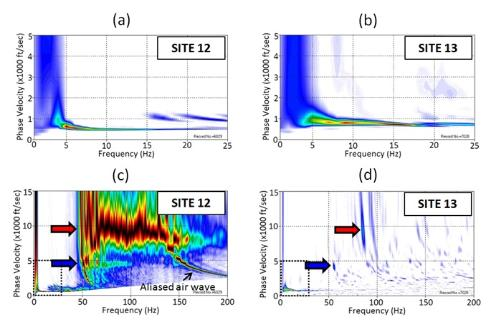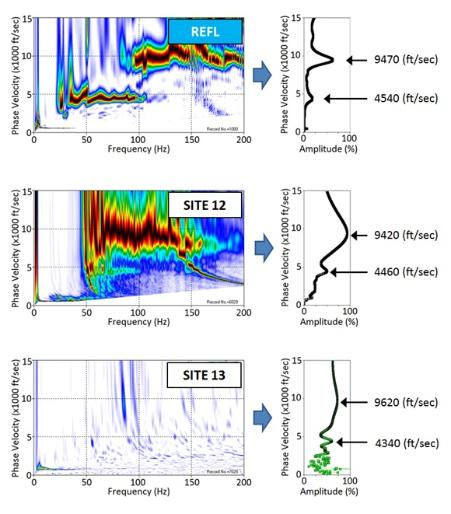(Above) Figure 4.  Dispersion images processed from field MASW records obtained from two
wind-turbine sites, (a) site 12 and (b) site 13.  Broad-range dispersion images for the two records
the range used in (a) and (b).

(Right) Figure 5.  Illustration of the frequency-summation (FRQ-Sum) method applied to the synthetic
(REFL) and the MASW records from sites 12 and 13.
synthetic record in Figure 1(b) using a broad range in frequency
and phase velocity.  The portion highlighted at lower left indicates
for the model in Figure 1(a) in the fundamental-mode (M0) and fifty
higher modes (M1-M50).